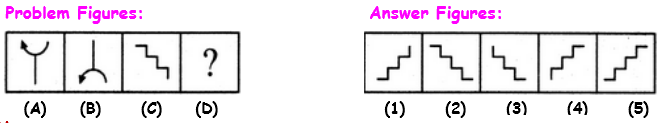# Analogy Interview Questions and Answers Part 1

## 1.Definition:

A comparison between one thing and another, typically for the purpose of explanation or clarification.

## Direction to solve:

Each of the following questions consists of two sets of figures. Figures A, B, C and D constitute the Problem Set while figures 1, 2, 3, 4 and 5 constitute the Answer Set.,
There is a definite relationship between figures A and B. Establish a similar relationship between figures C and D by selecting a suitable figure from the Answer Set that would replace the question mark (?) in fig. (D).

## 2.Select a suitable figure from the Answer Figures that would replace the question mark (?).1. 1
2. 2
3. 3
4. 4